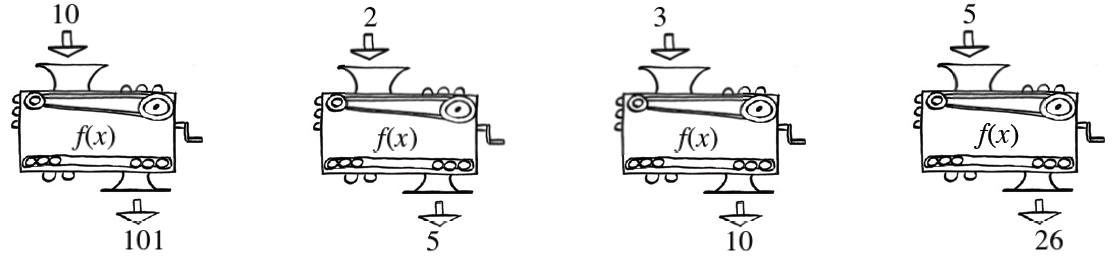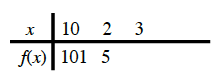### Home > INT3 > Chapter Ch2 > Lesson 2.2.4 > Problem2-98

2-98.

Each of the following pictures shows how the same machine changes the given $x$‑value into a corresponding $f(x)$-value. What is the equation for this machine? Homework Help ✎Copy and complete the table for the function.$f(x)=x^2+1$